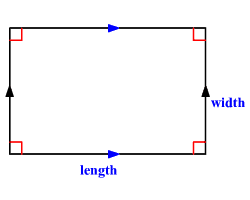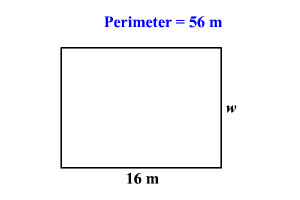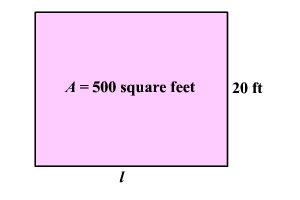# Word Problems: Area and Perimeter of a Rectangle

A rectangle is a parallelogram with four right angles. All rectangles are also parallelograms, but not all parallelograms are rectangles.The perimeter $P$ of a rectangle is given by the formula, $P=2l+2w$ , where $l$ is the length and $w$ is the width of the rectangle.

The area $A$ of a rectangle is given by the formula, $A=lw$ , where l is the length and $w$ is the width.

You will often encounter word problems where two of the values in one of these formulas are given, and you are required to find the third.

Example 1:

The perimeter of a rectangular pool is $56$ meters. If the length of the pool is $16$ meters, then find its width.

Here the perimeter and the length of the rectangular pool are given. We have to find the width of the pool.The perimeter $P$ of a rectangle is given by the formula, $P=2l+2w$ , where $l$ is the length and $w$ is the width of the rectangle.

Given that, the perimeter is $56$ meters and the length is $16$ meters. So, substitute these values into the formula.

$56=2\left(16\right)+2w$

Simplify.

$56=32+2w$

Subtract $32$ from both sides.

$24=2w$

Divide each side by $2$ .

$12=w$

Therefore, the width of the rectangular pool is $12$ meters.

Example 2:

The area of a rectangular fence is $500$ square feet. If the width of the fence is $20$ feet, then find its length.

Here the area and the width of the rectangular fence are given. We have to find the length of the fence.The area $A$ of a rectangle is given by the formula, $A=lw$ , where $l$ is the length and $w$ is the width.

Given that, the area is $500$ square feet and the width is $20$ feet. So, substitute these values into the formula.

$500=l×20$

Divide each side by $20$ to isolate $l$ .

$25=l$

Therefore, the length of the rectangular fence is $25$ feet.